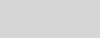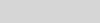# Use PowerShell to Easily Convert Decimal to Binary and BackDr Scripto

Summary: Learn how to use Windows PowerShell to easily convert decimal to binary and back, and simplify custom subnet mask calculations.

Microsoft Scripting Guy Ed Wilson here. Today is 63. Yep, that is right, today is 63. That is what you get when you see 11 11 11 represented as binary. This appears here.

 32 16 8 4 2 1 1 1 1 1 1 1

PS C:\> 1+2+4+8+16+32

63

A long time ago in this same galaxy, I wrote a VBScript function that would accept a binary number such as 111111 and translate it to a decimal value. It was a fun exercise, but it was also a bit complex. I had to get the length of the string, break it down piece by piece by position, calculate the value of that position, keep a running total, and loop back again. Now, I could translate that VBScript into Windows PowerShell, but that would be both a waste of time as well as downright misleading. This is because in Windows PowerShell, I have direct access to the .NET Framework Convert class. The Convert class resides in the system namespace, and it contains a large number of static methods for converting one type of thing to another. Well, okay, it contains 25 static methods and properties (I can use the Get-Member cmdlet and the Measure-Object cmdlet to obtain this information):

PS C:\> [convert] | gm -s | measure

Count    : 25

Average  :

Sum      :

Maximum  :

Minimum  :

Property :

The Convert class is well documented on MSDN, but in reality, the information obtained via the Get-Member cmdlet is usually enough information to make the conversion. For example, I can put the Convert class in square brackets, pipe it to Get-Member, use the static switch to retrieve static members, list the exact method I want to use, and send it to the Format-List cmdlet. This will show the method and all the associated overloads. This command sounds complicated, but it is really simple. The command is shown here:

[convert] | gm -s toint32 | fl *

The command and associated output are shown in the following figure.Hmm, it looks like there is a static method called ToInt32 that will accept a string value, so to use this method to convert a binary number, all I need to do is to pass the string and the number base (which is base 2 for the case of binary number conversion). The command shown here translates today’s date, 111111:

[convert]::ToInt32(“111111”,2)

The command and associated output are shown here.I can use the following script to translate a binary formatted subnet mask into decimal format:

\$a=\$i=\$null

“11111111”,”11111111″,”11111000″,”00000000″ |

% {

\$i++

[string]\$a += [convert]::ToInt32(\$_,2)

if(\$i -le 3) {[string]\$a += “.”}

}

\$a

ConvertBinarySubnetMaskToDecimal.ps1 demonstrates using the System.Convert .NET Framework class to convert from a binary number into decimal format. It uses a counter variable, \$i, to keep track of decimal placement. As each number passes over the pipeline, I store the converted value in the \$a variable. This script could easily be converted into a function if this were a task that is normally performed. The complete text of this script appears on the Scripting Guys Script Repository.

The flip side of the coin is translating a decimal number into binary format. To convert a decimal number into binary format, I once again use the Convert class, but this time, I use the ToString method. The syntax is similar to that of the ToInt32 method. The command shown here converts the decimal number 15 into a binary number.

[convert]::ToString(15,2)

The command and associated output appear here.

PS C:\> [convert]::ToString(15,2)

1111

After I know I can do that, I can also write a quick script to convert a decimal subnet mask to binary representation. This script is shown here:

\$a=\$i=\$null

“255”,”255″,”128″,”0″ |

% {

\$i++

[string]\$a += [convert]::ToString([int32]\$_,2)

if(\$i -le 3) {[string]\$a += “.”}

}

\$a

ConvertDecimalSubnetMaskToBinary.ps1 demonstrates using the System.Convert .NET Framework class to convert from a decimal number into binary format. It uses a counter variable, \$i, to keep track of decimal placement. As each number passes over the pipeline, I store the converted value in the \$a variable. This script could easily be converted into a function if this were a task that is normally performed. The complete text of this script appears on the Scripting Guys Script Repository.

Join me tomorrow as I introduce Guest Blogger Chris Walker who will talk about using Windows PowerShell to manage SharePoint profiles. It is a really good article, and I am certain you will enjoy it. Until then, see ya.

I invite you to follow me on Twitter and Facebook. If you have any questions, send email to me at scripter@microsoft.com, or post your questions on the Official Scripting Guys Forum. See you tomorrow. Until then, peace.

Ed Wilson, Microsoft Scripting Guy